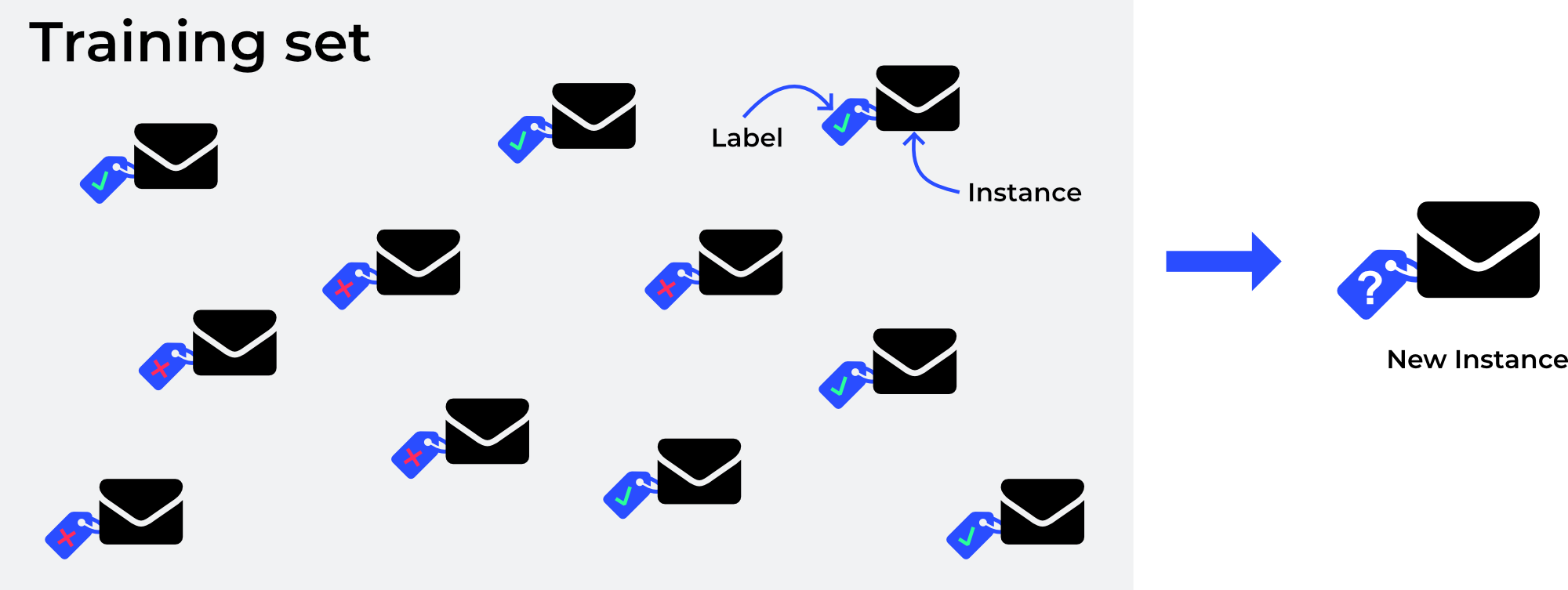## Introduction to supervised learning

Date: 25 June 2019

In this knowledge byte, we will be introducing the basic principles behind supervised learning, a common type of machine learning. If you haven't read our introduction article yet, you can find it here - But, what is machine learning actually?

Before we get into the specifics of supervised learning, we need to introduce some basic terms first.

Model - In machine learning, a model is the mathematical representation of a (real-world) process. An example would be a model that detects whether an email should be categorised as spam or ham (not spam).

Training data - In order to create a model, we need to hand a set of training data to a machine learning algorithm for it to learn. An algorithm needs this historical data to create the set of rules on which its future decisions will be based. Depending on the size of the available data set, this set is further split into a cross-validation and test set to validate and refine the chosen model.

#### Supervised learning

In a supervised learning case, the training data that is given to a machine learning algorithm includes the desired solutions, called labels. These solutions are often the actual outcomes of the process that the model represents. In the case of email spam detection, the training data includes a column indicating whether or not each email was spam or ham. These labels are provided through human input, meaning that if you mark an email as spam, you will put a label on a specific email.A spam filter is an example of a very typical type of supervised learning, classification. It is trained with many labeled example emails and has to learn from this experience to properly classify new emails.

A classification task can be achieved using different algorithms like k-Nearest Neighbour or logistic regression. While k-Nearest Neighbours (KNN) can be used for classification and regression problems, it is mostly used for classification. This algorithm will take a specific amount of data points “k”, which are closest to the new data point, to make a prediction. Using logistic regression, the model uses a function like the sigmoid function (S-shaped) to map predictions to probabilities. We then select a threshold or tipping point above which we will classify new instances into true or false.

Next to classification tasks, regression is another typical machine learning task. Regression is used to predict a target numeric value, for example the price of a house based on a set of features (e.g. number of rooms, location or square meters). As with a spam filter, we need a set of historical data to train this model. This could be a set of all houses sold in Amsterdam over the past ten years. A regression algorithm (e.g. a linear regression algorithm) would then, based on the available features, predict the price of a house that is about to enter the market.Other types of supervised learning - There are also other types of supervised learning that all have their own strengths and weaknesses like a support vector machine (SVM), decision trees and random forests as well as neural networks. Neural networks can also be used for unsupervised learning and we will go into way more detail about them in one of our upcoming articles, so stay posted.

Next week, we will look into unsupervised learning. In these tasks, there are no solutions (labels) available, making the creation of precise models more difficult. Make sure to subscribe to our LinkedIn page so you never miss a new article.##### Author: Jonas Weidner

Co-Founder and CTO

Jonas has a broad technical background in Mechanical Engineering, Industrial Design and Software Development.